Editor Version ×
Standard1.Easy to use and quick to get started

2.The process supports design scales of 300 devices or 1000 pads

3.Supports simple circuit simulation

4.For students, teachers, creators

Profession1.Brand new interactions and interfaces

2.Smooth support for design sizes of over 30,000 devices or 100,000 pads

3.More rigorous design constraints, more standardized processes

4.For enterprises, more professional usersStd edition JLCPCB -How to Read A Circuit Diagram

No Profile

Published Time: 2020-06-16 11:43:33
• 657
• 0
• 0
Description

This article is mainly for Electronic beginners for [PCB Design](PCB Design) and [DIY PCB Board](DIY PCB Board) or Electronic products.

JLCPCB \$2 for 2-Layer PCBs & \$5 for 4-Layer PCBs: https://jlcpcb.com

A circuit diagram usually has dozens or even hundreds of components, their connections crisscrossing, form changes, beginners often do not know where to start, how to read it. In fact, the electronic circuit itself has very strong regularity, no matter how complex the circuit, after analysis, it can be found that it is composed of a few unit circuits.No matters how complex the circuit is, it can be found after analysis that it is also composed of a few unit circuits. Therefore, beginners as long as the first familiar with the common basic unit circuit, and then learn to analyze and decompose the circuit ability, understand the general circuit diagram should be not difficult. According to the function of the unit circuit, they can be divided into several categories, and there are many kinds in each category. There are probably several hundred kinds of all unit circuits. Let's choose the most commonly used basic unit circuit to introduce. Let's start with the power circuit.

1. Graphical symbols The graphic symbol is the main body of the circuit diagram. Figure 1 shows the circuit diagram of the wireless microphone, in which various graphic symbols represent each component of the wireless microphone. For example, small rectangles represent resistors, two short bars represent capacitors, and continuous semicircles represent inductors. Each component graphic symbol is connected with the line, can reflect the wireless microphone's circuit structure, that is, constitute the wireless microphone's circuit diagram.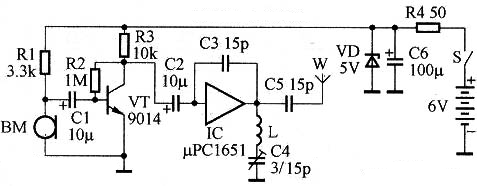1. Text symbols The text symbol is an important part of the circuit diagram. To further emphasize the nature of graphic symbols, but also for analysis, understanding, and elaboration For the convenience of the circuit diagram, the text symbol of each component shall be marked beside the graphic symbol of each component. For example, "R" means resistor, "C" means capacitor, "L" means inductor, "VT" means transistor, "IC" means integrated circuit, etc.

2. Annotation characters Annotation character is also an important part of the circuit diagram, which is used to explain the numerical size or specific model of components. In figure 1, for example, by commenting characters we can know, the value of resistor R1 Ω 3.3 k, the value of capacitor C1 is 10 mu F, transistor VT models for 9014, the model of integrated circuit IC for mu PCl651, etc.

1. The signal processing flow direction of circuit diagram The direction of the signal processing flow in the circuit diagram is generally from left to right. Each unit circuit that successively processes the signal is arranged in the direction from left to right, which is the most common arrangement form. For example, the circuit diagram of a wireless microphone is shown in Figure 1. From left to right are the voice signal reception (BM), audio amplification (VT), high-frequency oscillation and modulation (IC), and other unit circuits.

2. Cable connection Connection wires between components are represented by solid lines in the circuit diagram.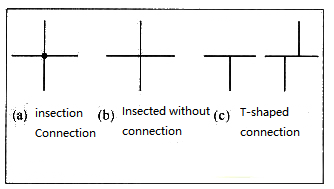1. Power cord and ground cable In the circuit diagram, the power lead is usually arranged above the component and the ground wire is arranged below the component, as shown in Figure 3(a). In some circuit diagrams, instead of connecting all the ground wires together, isolated grounding symbols are used instead, as shown in Figure 3(b), which should be understood as connecting all the ground wires together.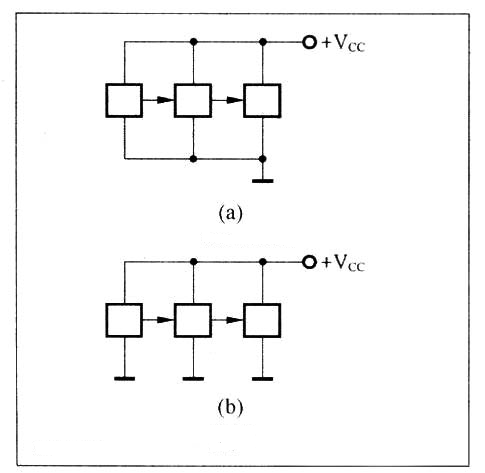and as picture 4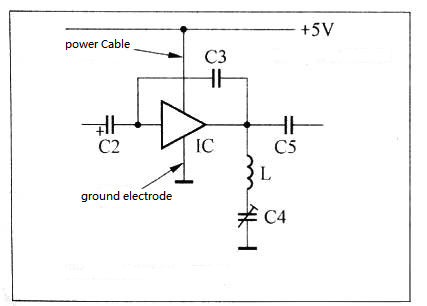1. Representation of integrated circuits Internal circuits of integrated circuits are generally very complex and consist of several unit circuits and many components. However, an INTEGRATED circuit is usually treated as a component in a circuit diagram. Therefore, the internal circuit of an INTEGRATED circuit is not drawn in almost all circuit diagrams but is represented by a rectangular or triangular block diagram. Integrated amplifiers, voltage comparators, etc. are conventionally represented by triangular block diagrams, while other integrated circuits are conventionally represented by rectangular block diagrams, as shown in FIG. 5. The left side is the input end and the right side is the output end.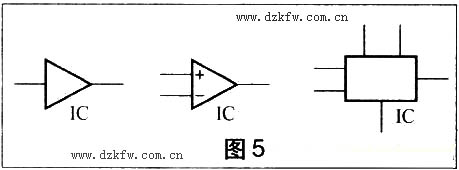The signal is converted into an electrical signal. (2) The audio amplifier circuit composed of transistor VT, etc., functions to amplify the audio signal output by BM. (3) High-frequency oscillation and modulation circuit composed of IC, etc., whose function is to generate high-frequency carrier frequency signal and complete frequency modulation.

1. Judge the direction of the signal processing flow

According to the overall function of the circuit diagram, the direction of the signal processing flow of the circuit diagram can be judged by finding the total input end and the total output end of the whole circuit circle. The function of the wireless microphone is to modulate the voice signal to the high-frequency signal and transmit it out. In the circuit diagram in Figure 1, the microphone BM is the total input end and the antenna W is the total output end. The direction from the total input end to the total output end is the signal processing flow direction. Figure 1 shows the direction from left to right.

1. Divide the unit circuit Generally speaking, transistors and integrated circuits are the core components of each unit circuit. Therefore, we can divide the circuit diagram into several unit circuits according to the direction of signal processing flow, and draw the circuit principle block diagram accordingly. Block diagrams help us to master and analyze circuit diagrams.

1 The circuit can be decomposed into three-unit circuits: (1) A voice signal receiving circuit composed of electret microphone BM, etc., whose function is to transfer voice Through the above two steps of analysis, we have a basic understanding of the wireless microphone circuit, can compare figure 1 circuit diagram and figure 6 block diagram, the principle of wireless microphone circuit system analysis. The working process of the circuit is as follows: after the voice signal is received and converted into an electrical signal by the electret microphone BM, it is input to the VT base of the transistor through the coupling capacitance C1. R1 is the load resistance of BM. The transistor VT and so on constitute the voltage amplifier, after the amplification of the audio signal coupled by C1, the output is coupled by C2. R2 is the base resistance and R3 is the collector resistance. Integrated circuit IC and so on constitute a high-frequency oscillator, the oscillation frequency is determined by L, C4 series resonance loop, C4 is the fine-tuning capacitor, used to adjust the oscillation frequency. C3 is the feedback capacitance. The frequency modulation of the high-frequency oscillation signal is carried out by the audio signal coupled with C2, and the frequency modulation signal is coupled to the antenna W and emitted.

1. Analyze dc power supply circuit The power supply is usually arranged on the right side and the DC supply circuit is arranged from right to left. In Figure 1, the dc power supply of the whole circuit is 6V battery, R4, C6, and the voltage regulator DIODE VD constitute the voltage regulator circuit to improve the stability of the circuit. S is the power switch.
design drawing
schematic diagram
1 /
PCB
1 /
Empty
BOM
Empty
Project Attachments
Empty
Add to album ×

reminder ×

Do you need to add this project to the album?• 0755 - 2382 4495
• 153 6159 2675

• 立创EDA微信号

easyeda• QQ交流群

664186054• 立创EDA公众号

lceda-cn